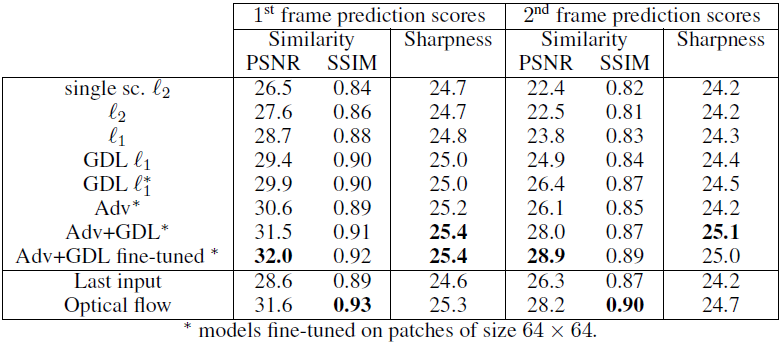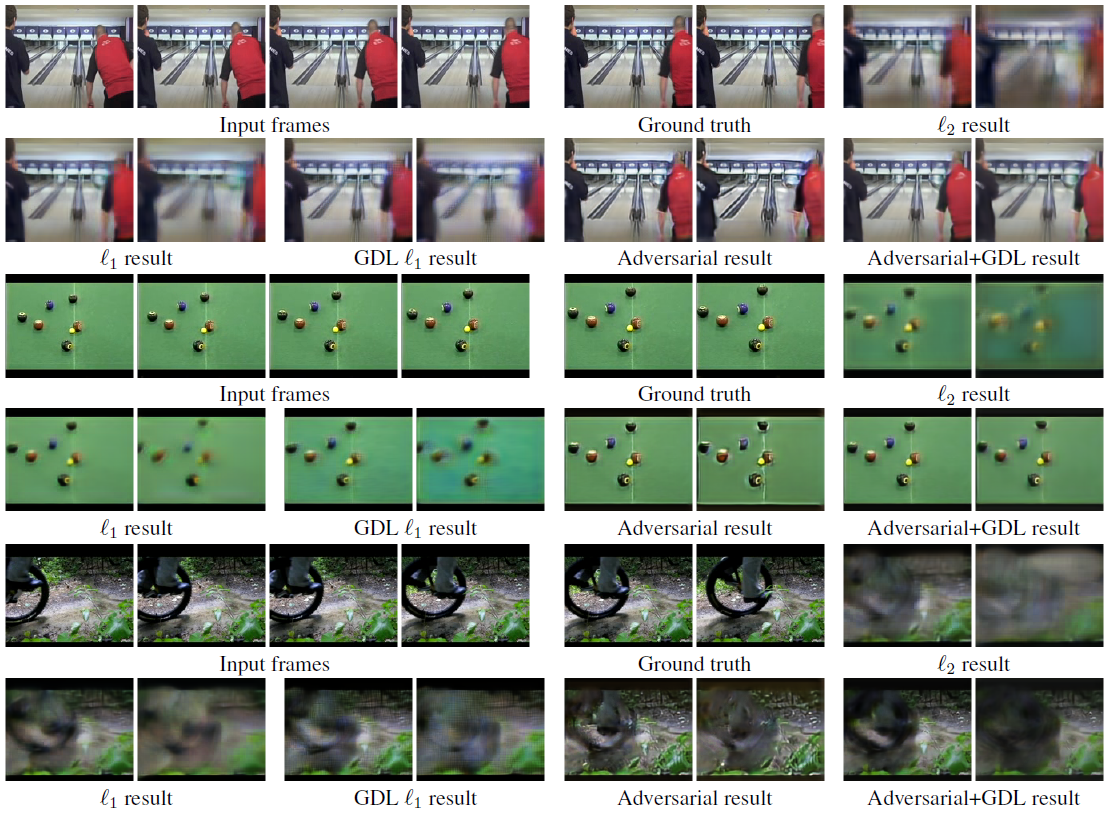# Review — Deep Multi-Scale Video Prediction Beyond Mean Square Error

## Video Frame Extrapolation, Generate Future Frames

Deep Multi-Scale Video Prediction Beyond Mean Square Error
Mathieu ICLR’16, by New York University, and Facebook Artificial Intelligence Research
2016 ICLR, Over 1700 Citations (Sik-Ho Tsang @ Medium)
Video Frame Extrapolation

• A CNN is proposed to generate future frames given an input sequence.
• To deal with the inherently blurry predictions obtained from the standard Mean Squared Error (MSE) loss function, three feature learning strategies are proposed: a multi-scale architecture, an adversarial training method, and an image gradient difference loss function.
• This is a paper from Prof. Yann LeCun research group.

# Outline

1. Proposed Network Architecture
2. Multi-Scale Architecture
4. Image Gradient Difference Loss (GDL)
5. Experimental Results

# 1. Proposed Network Architecture

• Let Y={Y1, …, Yn} be a sequence of frames to predict from input frames X={X1, …, Xm}.
• A LeNet-like network architecture G is trained to predict one or several concatenated frames Y from the concatenated frames X by minimizing a distance.
• where G(X) is the predicted frame and lp can be l1 or l2 norm.

However, convolutions only account for short-range dependencies. Also, using an l2 loss, and to a lesser extent l1, produces blurry predictions.

# 2. Multi-Scale Architecture

• A multi-scale version of the model is used.
• Let s1, …, sN scales be the sizes of the inputs of our network. Typically, in the experiments, s1=4×4, s2=8×8, s3=16×16 and s4=32×32.
• Let uk be the upscaling operator toward size sk, and let Xik, Yik denote the downscaled versions of Xi and Yi of size s.

This solve the problem of short-range dependencies.

# 3. Adversarial Training

• (Please feel free to read GAN for adversarial training.)
• The generator G is trained with a combined loss composed of the of the adversarial loss and the Lp loss:
• where LGadv is:
• The discriminator D to classify the input (X, Y) into class 1 and the input (X, G(X)) into class 0.

This solve the problem of blurry predictions.

# 4. Image Gradient Difference Loss (GDL)

• GDL is proposed to sharpen the image prediction by penalizing the differences of image gradient predictions in the generative loss function:
• And the total loss is:

This solve the problem of blurry predictions.

# 5. Experimental Results

• Two configurations are evaluated:
1. 4 input frames to predict one future frame. To generate further in the future, the model is applied recursively by using the newly generated frame as an input.
2. 8 input frames are used to produce 8 frames simultaneously.Comparison of the accuracy of the predictions on 10% of the UCF101 test images

The GDL and adversarial predictions are leading to further gains, and finally the combination of the multi-scale, l1 norm, GDL and adversarial training achieves the best PSNR, SSIM and Sharpness difference measure.Results on 3 video clips from Sport1m

As seen, the proposed Adversarial+GDL obtains more clearer results.

## References

[2016 ICLR] [Mathieu ICLR’16]
Deep Multi-Scale Video Prediction Beyond Mean Square Error

https://cs.nyu.edu/~mathieu/iclr2016.html

## Video Frame Interpolation/Extrapolation

2016 [Mathieu ICLR’16] 2017 [AdaConv] [SepConv] 2020 [DSepConv] 2021 [SepConv++]

--

--